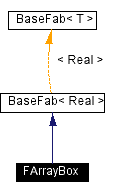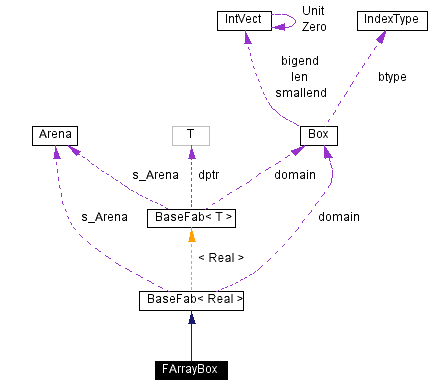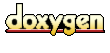Main Page   Namespace List   Class Hierarchy   Alphabetical List   Compound List   File List   Compound Members   File Members

# FArrayBox Class Reference

#include <FArrayBox.H>

Inheritance diagram for FArrayBox:[legend]
Collaboration diagram for FArrayBox:[legend]
List of all members.

## Public Methods

FArrayBox ()
{\bf constructors and destructor}

FArrayBox (const Box &b, int ncomp)
void define (const Box &b, int ncomp)
FArrayBox (std::istream &is)
virtual ~FArrayBox ()
virtual Real norm (int p=2, int comp=0, int numcomp=1) const
{\bf norm functions}

virtual Real norm (const Box &subbox, int p=2, int comp=0, int numcomp=1) const
virtual Real sumPow (const Box &subbox, int p=2, int comp=0, int numcomp=1) const
Real min (int comp=0) const
{\bf min and max functions}

Real min (const Box &subbox, int comp=0) const
Real max (int comp=0) const
Real max (const Box &subbox, int comp=0) const
IntVect minIndex (int comp=0) const
IntVect minIndex (const Box &subbox, int comp=0) const
IntVect maxIndex (int comp=0) const
IntVect maxIndex (const Box &subbox, int comp=0) const

void abs ()
{\bf Arithmetic functions}

void abs (int comp, int numcomp=1)
void abs (const Box &subbox, int comp=0, int numcomp=1)
Real sum (int comp, int numcomp=1) const
Real sum (const Box &subbox, int comp, int numcomp=1) const
FArrayBox & invert (Real v)
FArrayBox & invert (Real v, const Box &subbox, int comp=0, int numcomp=1)
FArrayBox & invert (Real v, int comp, int numcomp=1)
FArrayBox & negate (const Box &subbox, int comp=0, int numcomp=1)
FArrayBox & negate (int comp, int numcomp=1)
FArrayBox & negate ()
FArrayBox & plus (Real r, const Box &b, int comp=0, int numcomp=1)
FArrayBox & plus (Real r, int comp, int numcomp=1)
FArrayBox & operator+= (Real r)
FArrayBox & operator+= (const FArrayBox &f)
FArrayBox & plus (const FArrayBox &src)
FArrayBox & plus (Real r)
FArrayBox & plus_real (Real r)
FArrayBox & plus (const FArrayBox &src, const Real &scale)
FArrayBox & plus (const FArrayBox &src, const Real &scale, int srccomp, int destcomp, int numcomp=1)
FArrayBox & plus (const FArrayBox &src, int srccomp, int destcomp, int numcomp=1)
FArrayBox & plus (const FArrayBox &src, const Box &subbox, int srccomp, int destcomp, int numcomp=1)
FArrayBox & plus (const FArrayBox &src, const Box &srcbox, const Box &destbox, int srccomp, int destcomp, int numcomp=1)
FArrayBox & operator-= (Real r)
FArrayBox & operator-= (const FArrayBox &f)
FArrayBox & minus (const FArrayBox &src)
FArrayBox & minus (const FArrayBox &src, int srccomp, int destcomp, int numcomp=1)
FArrayBox & minus (const FArrayBox &src, const Box &subbox, int srccomp, int destcomp, int numcomp=1)
FArrayBox & minus (const FArrayBox &src, const Box &srcbox, const Box &destbox, int srccomp, int destcomp, int numcomp=1)
FArrayBox & operator *= (Real r)
FArrayBox & mult (Real r)
FArrayBox & mult (Real r, int comp, int numcomp=1)
FArrayBox & mult (Real r, const Box &b, int comp=0, int numcomp=1)
FArrayBox & operator *= (const FArrayBox &f)
FArrayBox & mult (const FArrayBox &src)
FArrayBox & mult (const FArrayBox &src, int srccomp, int destcomp, int numcomp=1)
FArrayBox & mult (const FArrayBox &src, const Box &subbox, int srccomp, int destcomp, int numcomp=1)
FArrayBox & mult (const FArrayBox &src, const Box &srcbox, const Box &destbox, int srccomp, int destcomp, int numcomp=1)
FArrayBox & operator/= (Real r)
FArrayBox & divide (Real r)
FArrayBox & divide (Real r, int comp, int numcomp=1)
FArrayBox & divide (Real r, const Box &b, int comp=0, int numcomp=1)
FArrayBox & operator/= (const FArrayBox &src)
FArrayBox & divide (const FArrayBox &src)
FArrayBox & divide (const FArrayBox &src, int srccomp, int destcomp, int numcomp=1)
FArrayBox & divide (const FArrayBox &src, const Box &subbox, int srccomp, int destcomp, int numcomp=1)
FArrayBox & divide (const FArrayBox &src, const Box &srcbox, const Box &destbox, int srccomp, int destcomp, int numcomp=1)
Real get (const IntVect &iv, int comp) const
void set (const IntVect &iv, int comp, Real val)

## Protected Methods

virtual void performCopy (const BaseFab< Real > &src, const Box &srcbox, int srccomp, const Box &destbox, int destcomp, int numcomp)

## Constructor & Destructor Documentation

 FArrayBox::FArrayBox ( )
 {\bf constructors and destructor} Constructs an invalid FArrayBox with no memory.

 FArrayBox::FArrayBox ( const Box & b, int ncomp )

 FArrayBox::FArrayBox ( std::istream & is )  [explicit]
 Constructs FArrayBox by reading it from istream.

 virtual FArrayBox::~FArrayBox ( )  [virtual]
 The (virtual) destructor.

## Member Function Documentation

 void FArrayBox::abs ( const Box & subbox, int comp = 0, int numcomp = 1 )
 Modifies this FArrayBox by replacing eahc value with its absolute value, for components (comp: comp+numcomp-1) and within the subbox.

 void FArrayBox::abs ( int comp, int numcomp = 1 )
 Modifies this FArrayBox by replacing eahc value with its absolute value, for components (comp: comp+numcomp-1).

 void FArrayBox::abs ( )
 {\bf Arithmetic functions} Modifies this FArrayBox by replacing each value with its absolute value.

 void FArrayBox::define ( const Box & b, int ncomp )  [inline, virtual]
 Reimplemented from BaseFab< Real >.

 FArrayBox& FArrayBox::divide ( const FArrayBox & src, const Box & srcbox, const Box & destbox, int srccomp, int destcomp, int numcomp = 1 )

 FArrayBox& FArrayBox::divide ( const FArrayBox & src, const Box & subbox, int srccomp, int destcomp, int numcomp = 1 )

 FArrayBox& FArrayBox::divide ( const FArrayBox & src, int srccomp, int destcomp, int numcomp = 1 )

 FArrayBox& FArrayBox::divide ( const FArrayBox & src )
 Modifies this FArrayBox by pointwise division by the values in the argument FArrayBox. You might come to grief if the domains of the FArrayBoxes don't match. The same as the /= operator.

 FArrayBox& FArrayBox::divide ( Real r, const Box & b, int comp = 0, int numcomp = 1 )
 Modifies this FArrayBox by dividing all values by the scalar Real r. For given range of components and within given subbox.

 FArrayBox& FArrayBox::divide ( Real r, int comp, int numcomp = 1 )
 Modifies this FArrayBox by dividing all values by the scalar Real r. For given range of components.

 FArrayBox& FArrayBox::divide ( Real r )
 Modifies this FArrayBox by dividing all values by the scalar Real r.

 Real FArrayBox::get ( const IntVect & iv, int comp ) const [inline]

 FArrayBox& FArrayBox::invert ( Real v, int comp, int numcomp = 1 )
 Modifies this FArrayBox by replacing each value x with v/x. For given range of components.

 FArrayBox& FArrayBox::invert ( Real v, const Box & subbox, int comp = 0, int numcomp = 1 )
 Modifies this FArrayBox by replacing each value x with v/x. For given range of components and within given subbox.

 FArrayBox& FArrayBox::invert ( Real v )
 Modifies this FArrayBox by replacing each value x with v/x.

 int FArrayBox::maskEQ ( BaseFab< int > & mask, Real val, int comp = 0 ) const
 Computes mask array with value of 1 in cells where this FArrayBox has value equal to val, 0 otherwise. mask is resized by this function. The number of cells marked with 1 returned.

 int FArrayBox::maskGE ( BaseFab< int > & mask, Real val, int comp = 0 ) const
 Computes mask array with value of 1 in cells where this FArrayBox has value greater than or equal to val, 0 otherwise. mask is resized by this function. The number of cells marked with 1 returned.

 int FArrayBox::maskGT ( BaseFab< int > & mask, Real val, int comp = 0 ) const
 Computes mask array with value of 1 in cells where this FArrayBox has value greater than val, 0 otherwise. mask is resized by this function. The number of cells marked with 1 returned.

 int FArrayBox::maskLE ( BaseFab< int > & mask, Real val, int comp = 0 ) const
 Computes mask array with value of 1 in cells where this FArrayBox has value less than or equal to val, 0 otherwise. mask is resized by this function. The number of cells marked with 1 returned.

 int FArrayBox::maskLT ( BaseFab< int > & mask, Real val, int comp = 0 ) const
 {\bf mask functions} Computes mask array with value of 1 in cells where this FArrayBox has value less than val, 0 otherwise. mask is resized by this function. The number of cells marked with 1 returned.

 Real FArrayBox::max ( const Box & subbox, int comp = 0 ) const
 Returns the maximum value of given component of this FArrayBox in given subbox.

 Real FArrayBox::max ( int comp = 0 ) const
 Returns the maximum value of given component of this FArrayBox.

 IntVect FArrayBox::maxIndex ( const Box & subbox, int comp = 0 ) const

 IntVect FArrayBox::maxIndex ( int comp = 0 ) const
 Returns location of maximum value in given component of this FArrayBox.

 Real FArrayBox::min ( const Box & subbox, int comp = 0 ) const
 Returns the minimum value of given component of this FArrayBox in given subbox.

 Real FArrayBox::min ( int comp = 0 ) const
 {\bf min and max functions} Returns the minimum value of given component of this FArrayBox.

 IntVect FArrayBox::minIndex ( const Box & subbox, int comp = 0 ) const

 IntVect FArrayBox::minIndex ( int comp = 0 ) const
 Finds location of minimum value in given component of this FArrayBox.

 FArrayBox& FArrayBox::minus ( const FArrayBox & src, const Box & srcbox, const Box & destbox, int srccomp, int destcomp, int numcomp = 1 )

 FArrayBox& FArrayBox::minus ( const FArrayBox & src, const Box & subbox, int srccomp, int destcomp, int numcomp = 1 )

 FArrayBox& FArrayBox::minus ( const FArrayBox & src, int srccomp, int destcomp, int numcomp = 1 )

 FArrayBox& FArrayBox::minus ( const FArrayBox & src )
 Modifies this FArrayBox by pointwise subtraction of the values of the argument FArrayBox. You might come to grief if the domains of the FArrayBoxes don't match. The same as -= operator.

 FArrayBox& FArrayBox::mult ( const FArrayBox & src, const Box & srcbox, const Box & destbox, int srccomp, int destcomp, int numcomp = 1 )

 FArrayBox& FArrayBox::mult ( const FArrayBox & src, const Box & subbox, int srccomp, int destcomp, int numcomp = 1 )

 FArrayBox& FArrayBox::mult ( const FArrayBox & src, int srccomp, int destcomp, int numcomp = 1 )

 FArrayBox& FArrayBox::mult ( const FArrayBox & src )
 Modifies this FArrayBox by pointwise multiplication by the values in the argument FArrayBox. You might come to grief if the domains of the FArrayBoxes don't match. The same as the *= operator.

 FArrayBox& FArrayBox::mult ( Real r, const Box & b, int comp = 0, int numcomp = 1 )
 Modifies this FArrayBox by multiplying all values by the scalar Real r. For given range of components and within given subbox.

 FArrayBox& FArrayBox::mult ( Real r, int comp, int numcomp = 1 )
 Modifies this FArrayBox by multiplying all values by the scalar Real r. For given range of components.

 FArrayBox& FArrayBox::mult ( Real r )
 Modifies this FArrayBox by multiplying all values by the scalar Real r.

 FArrayBox& FArrayBox::negate ( )
 Modifies this FArrayBox by replacing each value with its additive inverse.

 FArrayBox& FArrayBox::negate ( int comp, int numcomp = 1 )
 Modifies this FArrayBox by replacing each value with its additive inverse. For given range of components.

 FArrayBox& FArrayBox::negate ( const Box & subbox, int comp = 0, int numcomp = 1 )
 Modifies this FArrayBox by replacing each value with its additive inverse. For given range of components and within given subbox.

 virtual Real FArrayBox::norm ( const Box & subbox, int p = 2, int comp = 0, int numcomp = 1 ) const [virtual]

 virtual Real FArrayBox::norm ( int p = 2, int comp = 0, int numcomp = 1 ) const [virtual]
 {\bf norm functions}

 FArrayBox& FArrayBox::operator *= ( const FArrayBox & f )
 Modifies this FArrayBox by pointwise multiplication of the values by the argument FArrayBox. You might come to grief if the domains of the FArrayBoxes don't match.

 FArrayBox& FArrayBox::operator *= ( Real r )
 Modifies this FArrayBox by multiplying all values by the scalar Real r.

 FArrayBox& FArrayBox::operator+= ( const FArrayBox & f )
 Modifies this FArrayBox by pointwise addition of the values of the argument FArrayBox. You might come to grief if the domains of the FArrayBoxes don't match.

 FArrayBox& FArrayBox::operator+= ( Real r )
 Modifies this FArrayBox by adding the scalar Real r to all values.

 FArrayBox& FArrayBox::operator-= ( const FArrayBox & f )
 Modifies this FArrayBox by pointwise subtraction of the values of the argument FArrayBox. You might come to grief if the domains of the FArrayBoxes don't match.

 FArrayBox& FArrayBox::operator-= ( Real r )
 Modifies this FArrayBox by subtracting the scalar Real r to all values. Note: use plus(-r) for more general operations.

 FArrayBox& FArrayBox::operator/= ( const FArrayBox & src )
 Modifies this FArrayBox by pointwise division of the values by the argument FArrayBox. You might come to grief if the domains of the FArrayBoxes don't match.

 FArrayBox& FArrayBox::operator/= ( Real r )
 Modifies this FArrayBox by dividing all values by the scalar Real r.

 virtual void FArrayBox::performCopy ( const BaseFab< Real > & src, const Box & srcbox, int srccomp, const Box & destbox, int destcomp, int numcomp )  [protected, virtual]
 Reimplemented from BaseFab< Real >.

 FArrayBox& FArrayBox::plus ( const FArrayBox & src, const Box & srcbox, const Box & destbox, int srccomp, int destcomp, int numcomp = 1 )

 FArrayBox& FArrayBox::plus ( const FArrayBox & src, const Box & subbox, int srccomp, int destcomp, int numcomp = 1 )

 FArrayBox& FArrayBox::plus ( const FArrayBox & src, int srccomp, int destcomp, int numcomp = 1 )

 FArrayBox& FArrayBox::plus ( const FArrayBox & src, const Real & scale, int srccomp, int destcomp, int numcomp = 1 )
 Modifies this FArrayBox by pointwise scaled addition of the argument FArrayBox (a[i] <- a[i] + scale * src[i]). Uses domain of the intersection of these two FArrayBoxes.

 FArrayBox& FArrayBox::plus ( const FArrayBox & src, const Real & scale )
 Modifies this FArrayBox by pointwise scaled addition of the argument FArrayBox (a[i] <- a[i] + scale * src[i]). Uses domain of the intersection of these two FArrayBoxes.

 FArrayBox& FArrayBox::plus ( Real r )
 Modifies this FArrayBox by adding the scalar Real r to all values.

 FArrayBox& FArrayBox::plus ( const FArrayBox & src )
 Modifies this FArrayBox by pointwise addition of the values of the argument FArrayBox. You might come to grief if the domains of the FArrayBoxes don't match. The same as += operator.

 FArrayBox& FArrayBox::plus ( Real r, int comp, int numcomp = 1 )
 Modifies this FArrayBox by adding the scalar Real r to all values. For given range of components.

 FArrayBox& FArrayBox::plus ( Real r, const Box & b, int comp = 0, int numcomp = 1 )
 Modifies this FArrayBox by adding the scalar Real r to all values. For given range of components and within given subbox.

 FArrayBox& FArrayBox::plus_real ( Real r )  [inline]

 void FArrayBox::set ( const IntVect & iv, int comp, Real val )  [inline]

 Real FArrayBox::sum ( const Box & subbox, int comp, int numcomp = 1 ) const

 Real FArrayBox::sum ( int comp, int numcomp = 1 ) const
 Returns sum of given component of FArrayBox.

 virtual Real FArrayBox::sumPow ( const Box & subbox, int p = 2, int comp = 0, int numcomp = 1 ) const [virtual]
 Returns sum of pow(fab[i,c],p): i in subbox, comp<=c=2 only

The documentation for this class was generated from the following file:
Generated on Thu Aug 29 11:07:41 2002 for Chombo&INS by1.2.16